# Class 11 RD Sharma Solutions – Chapter 6 Graphs of Trigonometric Functions – Exercise 6.3

• Last Updated : 08 May, 2021

### Question 1: y = sin2 x

Solution:

As we know that,

y = sin2 x =On shifting the origin at (0, 1/2), we get

X = x and Y =On substituting these values, we getThe maximum and minimum values of Y areandrespectively and shift it by 1/2 to the up.

As the equation in the form of y = – f(x), the graph become inverted of y = f(x)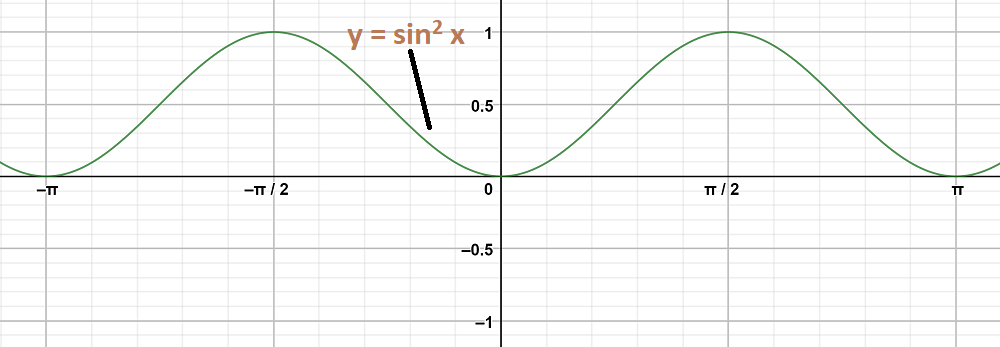### Question 2: y = cos2 x

Solution:

As we know that,

y = cos2 x =On shifting the origin at, we get

X = x and Y =On substituting these values, we getThe maximum and minimum values of Y areandrespectively and shift it by 1/2 to the up.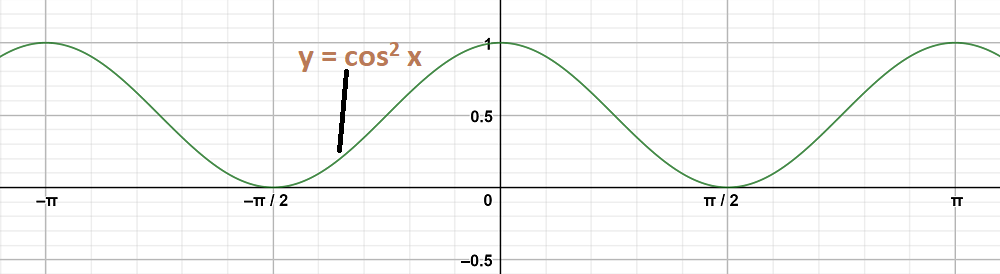### Question 3: y = sin2Solution:

To obtain this graph y-0 = sin2On shifting the origin at (,0), we get

X =and Y = y – 0

On substituting these values, we get

Y = sin2 X

First we draw the graph of Y = sin2 X and shift it by π/4 to the right.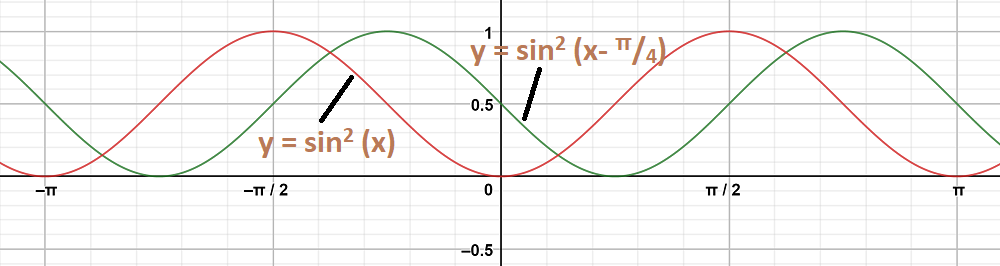### Question 4: y = tan 2x

Solution:

To obtain this graph y = tan 2x,

First we draw the graph of y = tan x and then divide the x-coordinates of the points where it crosses x-axis by 2.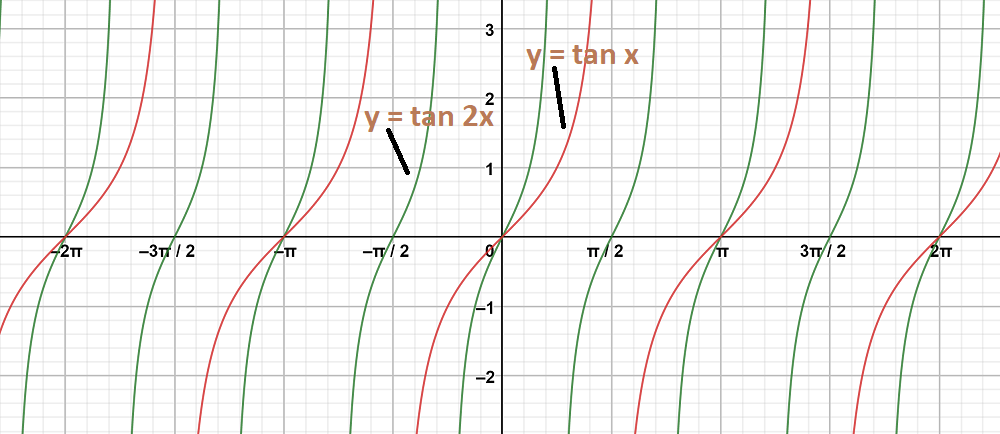### Question 5: y = 2 tan 3x

Solution:

To obtain this graph y = 2 tan 3x,

First we draw the graph of y = tan x and then divide the x-coordinates of the points where it crosses x-axis by 3.

Stretch the graph vertically by the factor of 2.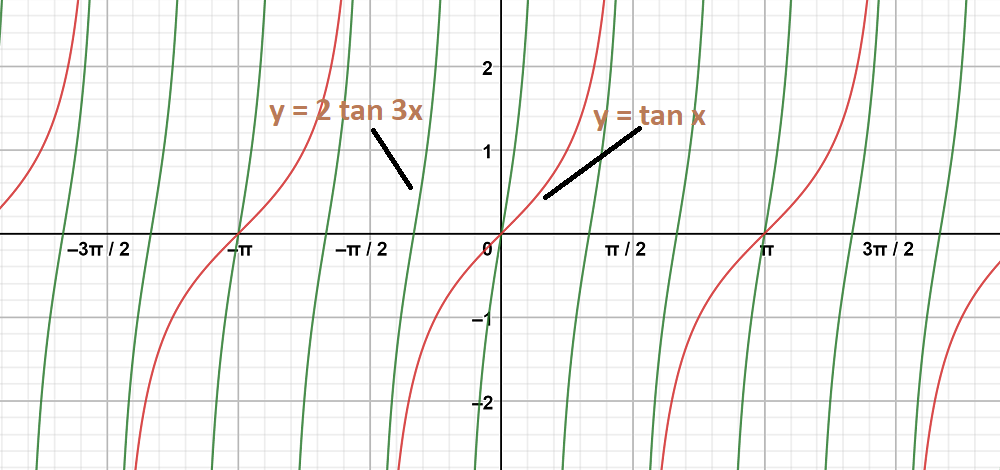### Question 6: y = 2 cot 2x

Solution:

To obtain this graph y = 2 cot 2x,

First we draw the graph of y = cot x and then divide the x-coordinates of the points where it crosses x-axis by 2.

Stretch the graph vertically by the factor of 2.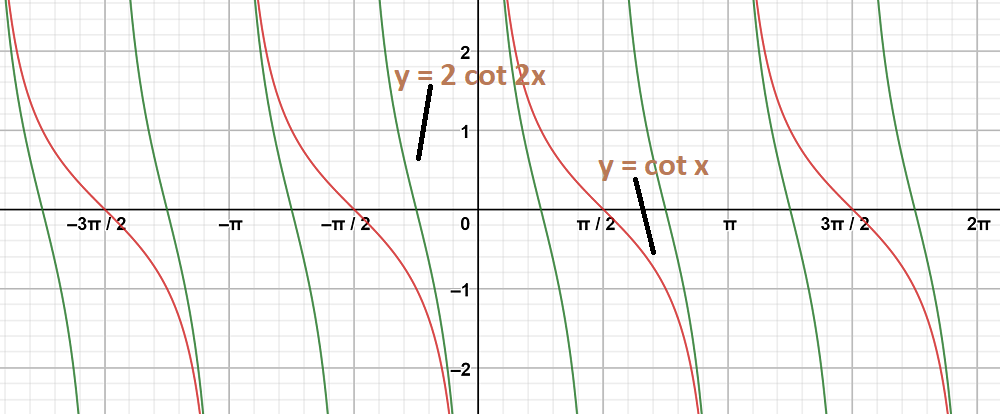### Question 7: y = cos 2x, y = cosSolution:

Graph 1:

y = cos2 x

As we know that,

y = cos2 x =On shifting the origin at (0, 1/2), we get

X = x and Y =On substituting these values, we getThe maximum and minimum values of Y areandrespectively and shift it by 1/2 to the up.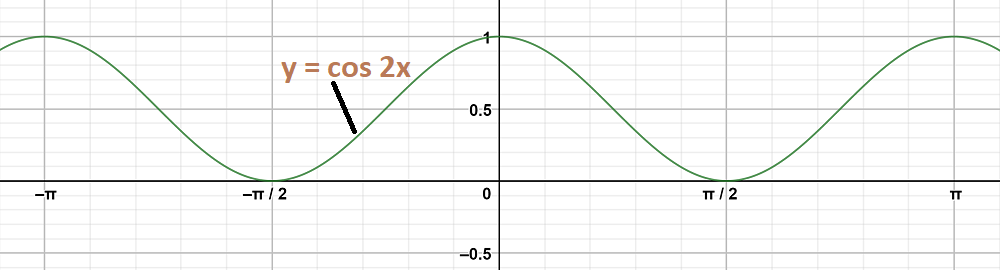Graph 2:

To obtain this graph y-0 = cos (2x-) = cos 2(x-)

On shifting the origin at (π/6, 0), we get

X =and Y = y – 0

On substituting these values, we get

Y = cos 2X

First we draw the graph of Y = cos 2X and shift it by π/6 to the right.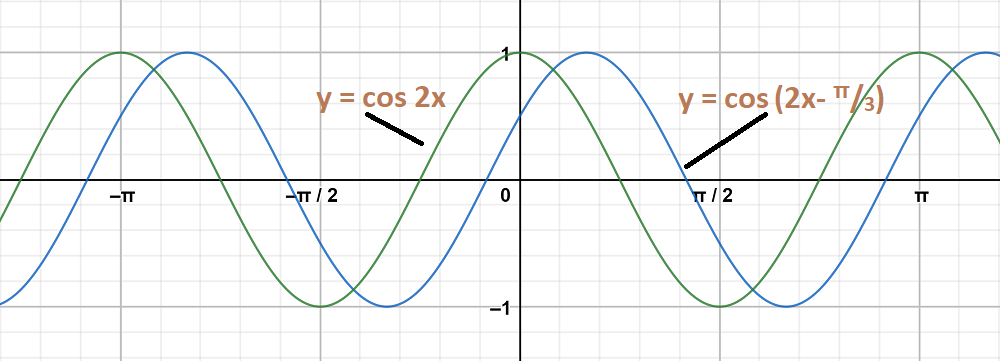The graph y = cos2 x and y = cosare on same axes are as follows: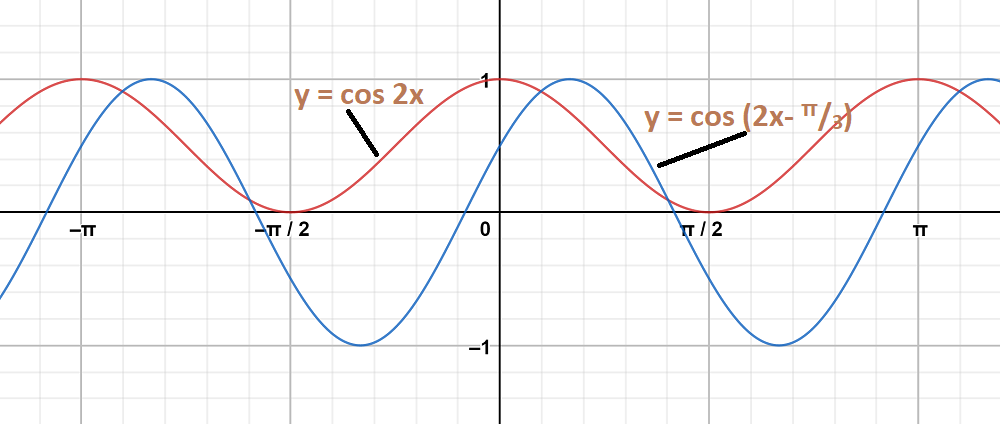### Question 8: y = sin2 x, y = sin x

Solution:

Graph 1:

y = sin2 x

As we know that,

y = sin2 x =On shifting the origin at (0,), we get

X = x and Y =On substituting these values, we getThe maximum and minimum values of Y areandrespectively and shift it by 1/2 to the up.

As the equation in the form of y = – f(x), the graph become inverted of y = f(x)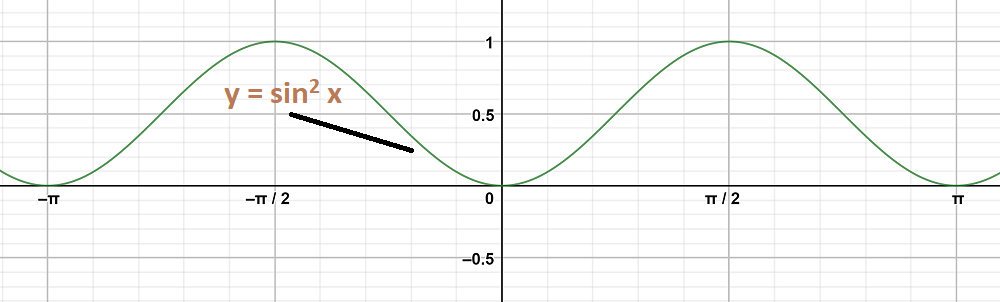Graph 2:

y = sin x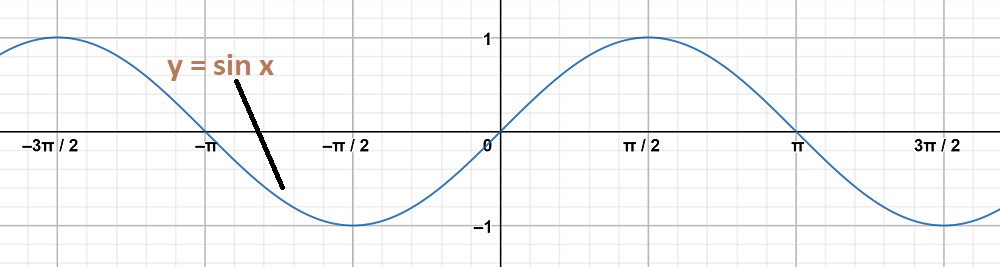The graph y = sin2 x and y = sin x are on same axes are as follows: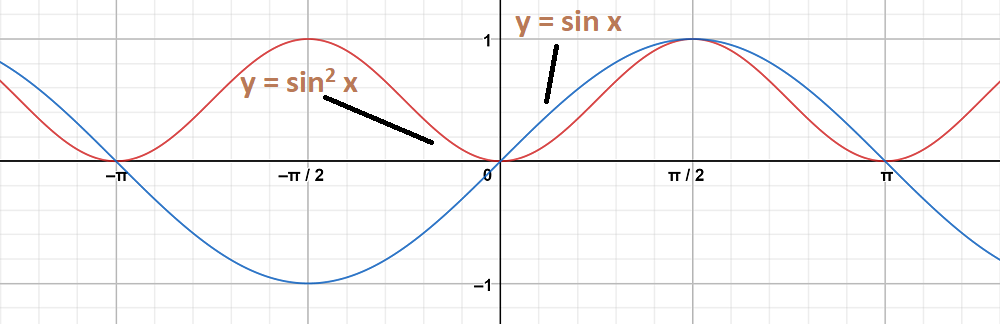### Question 9: y = tan x, y = tan 2x

Solution:

Graph 1:

y = tan x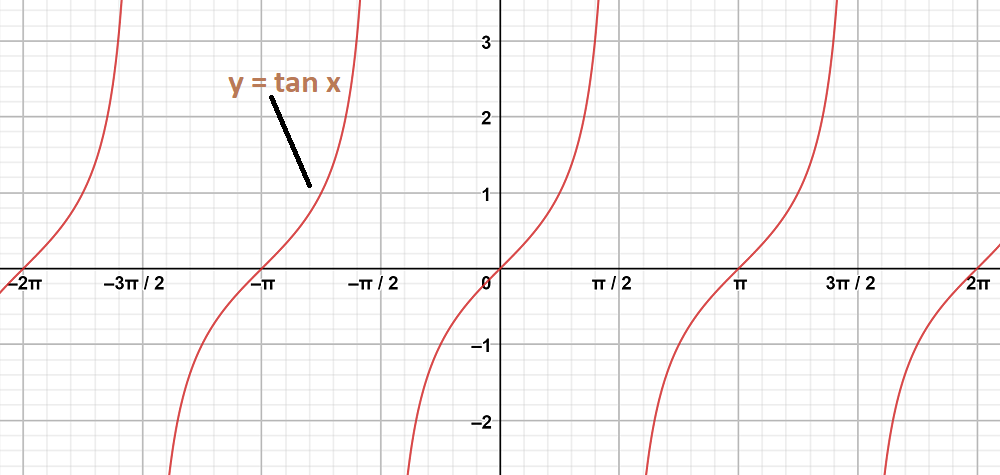Graph 2:

y = tan2 x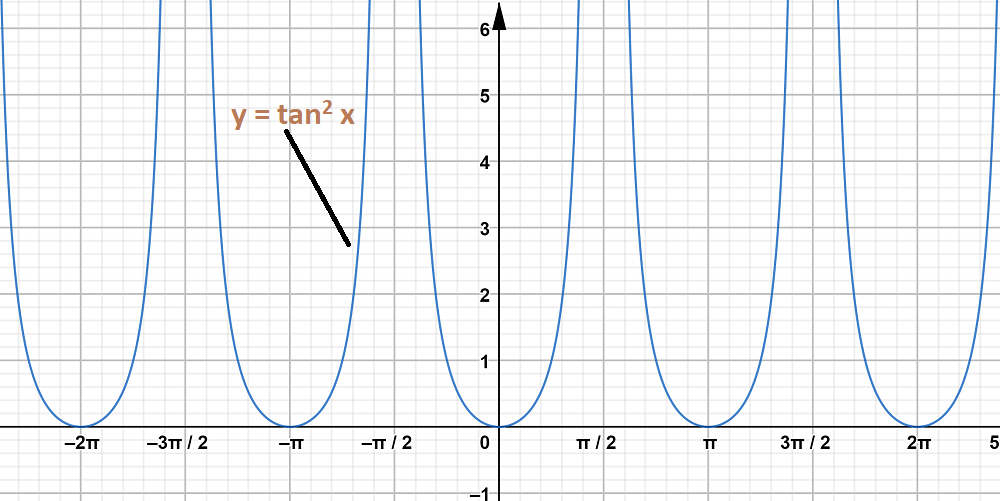The graph y = tan x and y = tan2 x are on same axes are as follows: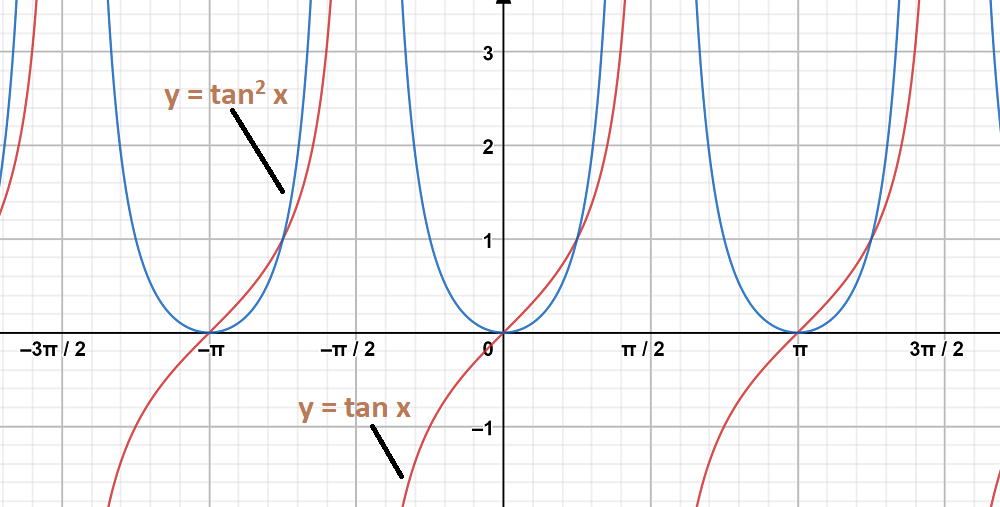### Question 10: y = tan 2x, y = tan x

Solution:

Graph 1:

To obtain this graph y = tan 2x,

First we draw the graph of y = tan x and then divide the x-coordinates of the points where it crosses x-axis by 2.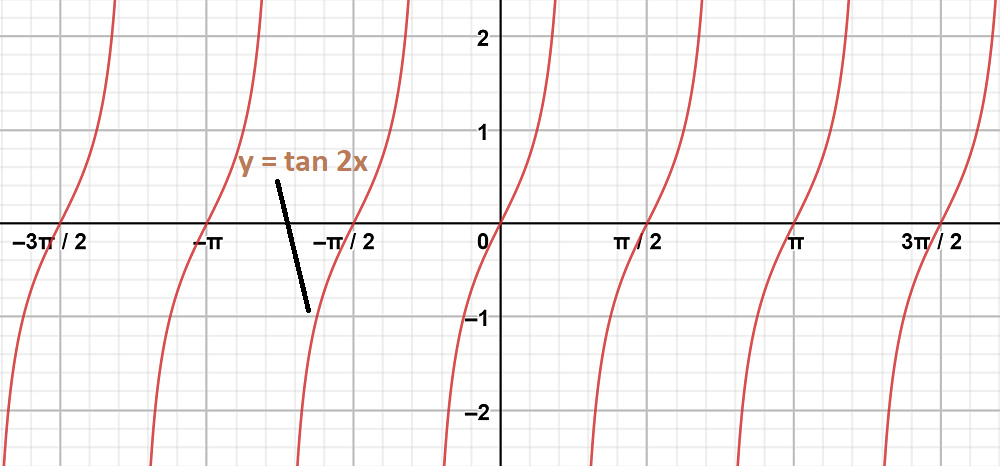Graph 2:

y = tan x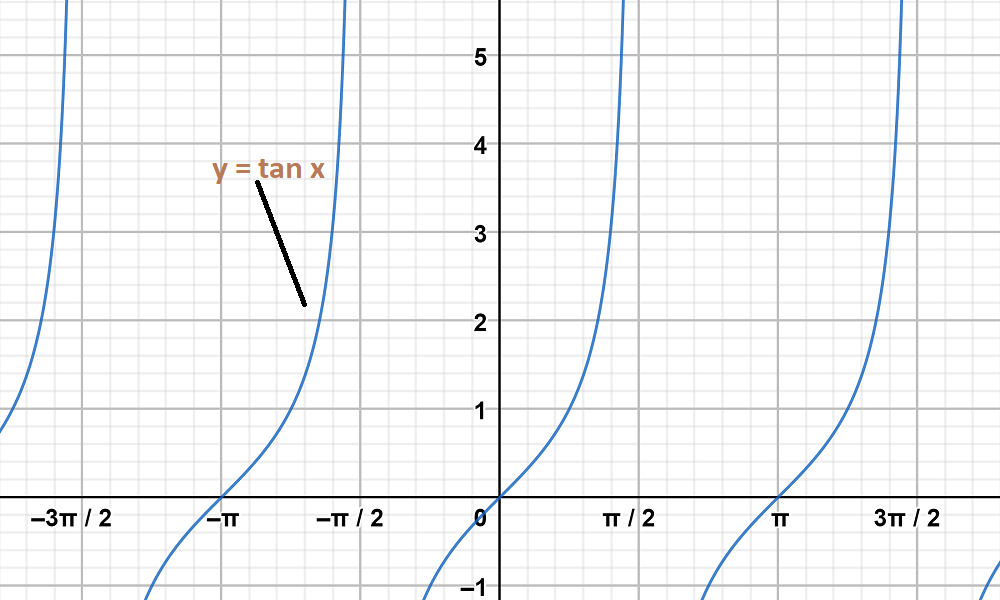The graph y = tan 2x and y = tan x are on same axes are as follows: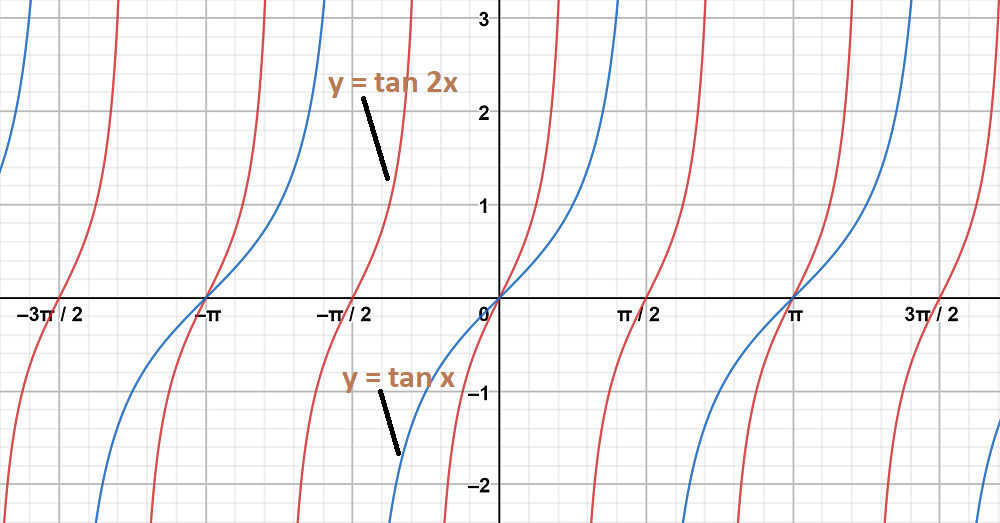My Personal Notes arrow_drop_up